Quadratic functions are used in math and science to model various natural phenomena (such as the height of falling objects).  However, there are some common questions about quadratic functions that it helps to know the answers to.

So, what is there to know about quadratic functions?  A quadratic function has 0, 1, or 2 x-intercepts, and it has the graph of a parabola that opens up or down, not a straight line or periodic function. A quadratic function on a restricted domain can have an inverse and also both a maximum & minimum. No quadratic function has a negative exponent.

Of course, there are lots of difference scenarios for the graphs of quadratic functions (parabolas).  However, we can classify these into a few cases without too much trouble.

In this article, we’ll talk about quadratic functions in the context of 8 common questions about them.  We’ll also look at some graphs to illustrate some of the ideas.

Let’s get started.

Quadratic functions raise lots of questions about the nature of their solutions, the appearance of their graphs, and their extreme (maximum and minimum values).

Let’s start off with a question concerning the solutions (or roots or zeros) of a quadratic function.

### Can A Quadratic Function Have No X-Intercepts?

It is possible for a quadratic function to have no x-intercepts (also called zeros) if the corresponding quadratic equation has no real solutions.  Remember that this happens when the discriminant (the term b2 – 4ac under the radical) in the quadratic formula is negative.

In this case, the two solutions to the quadratic equation are complex conjugates of the form a + bi and a – bi.  Since the solutions are complex with a nonzero imaginary part, they will not appear on a graph in the xy coordinate plane.

This means that the quadratic has no real roots. Graphically, this means there are two cases for a quadratic function f(x) with no x-intercepts:

• The parabola is always above the x-axis.  In this case, we must have a vertex above the x-axis and f’’(x) > 0, meaning f(x) is convex.
• The parabola is always below the x-axis.  In this case, we must have a vertex below the x-axis and f’’(x) < 0, meaning f(x) is concave.

You can see some examples of quadratic functions with no x-intercepts below.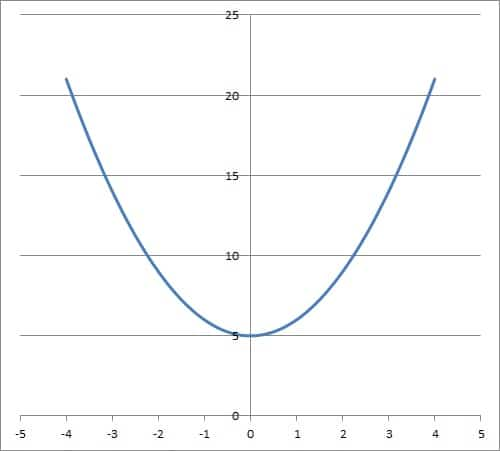The graph of the parabola y = x2 + 5 has no x-intercepts. It is always above the x-axis (the line y = 0).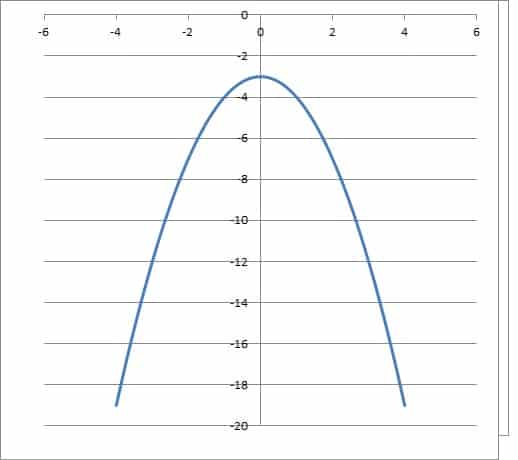The graph of the parabola y = -x2 – 3 has no x-intercepts. It is always below the x-axis

Note that there are two other cases for the x-intercepts of a quadratic function:

• A single x-intercept – in this case, the vertex rests on the x-axis, and the x-coordinate of the vertex is a double (repeated) root of the quadratic.  This also means the discriminant is zero (that is, b2 – 4ac = 0).  This quadratic has only one real solution.
• Two distinct x-intercepts – in this case, the vertex does not rest on the x-axis, and there are two roots of the quadratic: one to the left of the vertex, and one to the right of the vertex.  This also means the discriminant is positive (that is, b2 – 4ac > 0).  This quadratic has two real solutions.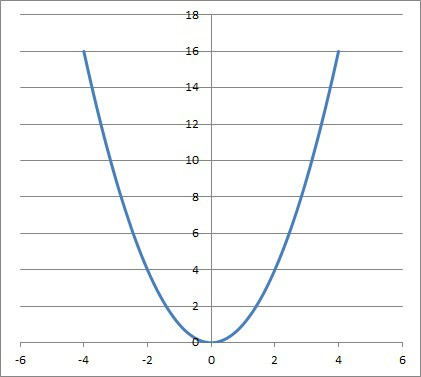The graph of the parabola y = x2 has one x-intercept. Its vertex rests on the x-axis (the line y = 0).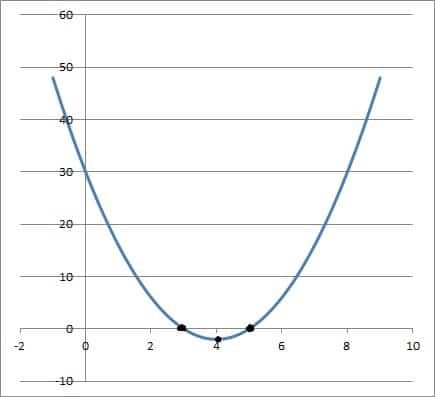The graph of the parabola f(x) = 2(x – 3)(x – 5) has two x-intercepts at x = 3 and x = 5. Its vertex is at x = 4 (halfway between the two zeros).

The graphic below summarizes the 3 possible cases for solutions of a quadratic equation, based on the sign of the discriminant.

### Can A Quadratic Function Be A Straight Line?

A quadratic function cannot be a straight line.  By definition, a quadratic function has the form

• f(x) = ax2 + bx + c

where a, b, and c are real numbers and a is not zero.A quadratic has the form ax2 + bx + c. The coefficients b and c may be zero, but a cannot be.

If we have the graph of a straight line, it has the form bx + c.  This implies a = 0, which means the graph is not a quadratic function.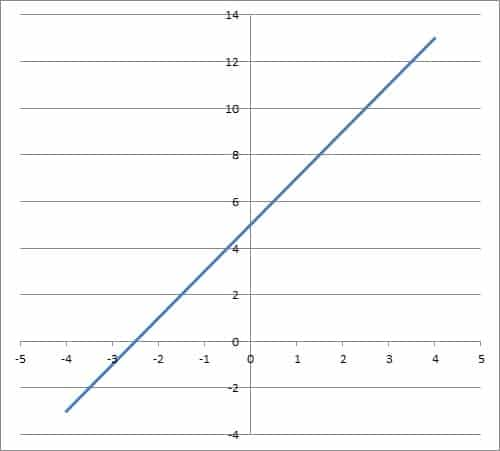The graph of the line y = 2x + 5. This is not a quadratic function, since a = 0 (that is, the x-squared term has a coefficient of zero).

### Can A Quadratic Function Have Three Zeros?

A quadratic function cannot have three zeros.  A quadratic function has exactly two zeros (or roots), which may be real or complex with nonzero imaginary parts.

A quadratic function has degree 2, which means that the highest power of x is 2 (in the ax2 term).  According to the Fundamental Theorem of Algebra, a degree n polynomial with complex coefficients has exactly n complex roots.

This means that a quadratic function (with degree n = 2) has exactly two roots.  We know the cases from earlier:

• Two distinct real roots – this occurs when the discriminant b2 – 4ac > 0.
• One repeated real root – also called a double root.  This occurs when the discriminant b2 – 4ac = 0.
• Two complex conjugate roots – this occurs when the discriminant b2 – 4ac < 0.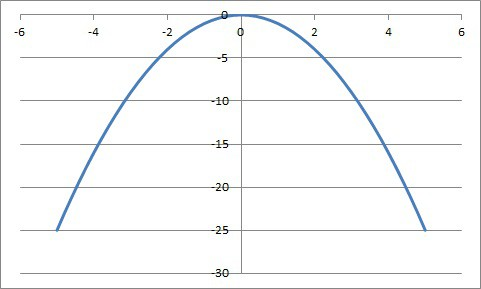This parabola has one repeated (double) real root, meaning that the discriminant is zero (and the vertex rests on the x-axis).

Remember that each real zero of a quadratic function represents a point where the graph intersects the x-axis (or the line y = 0).  These points are also known as x-intercepts.

### Can A Quadratic Function Be Periodic?

A quadratic function cannot be periodic.  At most, a quadratic function will repeat a given y value twice (due to the degree of a quadratic being 2; see the Fundamental Theorem of Algebra earlier in this article).

A function like f(x) = sin(x) is periodic because certain y values repeat indefinitely as we take increasing x values.  For example, sin(x) has a value of 0 for any multiple of pi: sin(0), sin(π), sin(2π), sin(3π), … are all zero.

This is also true for negative multiples of pi: sin(-π), sin(-2π), sin(-3π), … are all zero as well.

You can see the graph of a periodic function sine function below.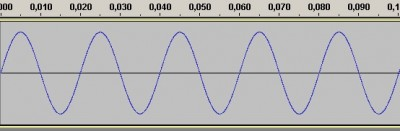A sine wave is periodic because some y values repeat indefinitely as we continue to increase (or decrease) the value of x.

### Can A Quadratic Function Have A Maximum & A Minimum?

A quadratic function can have a maximum and a minimum if its domain is restricted (at least one closed endpoint].  For example, consider the quadratic function f(x) = x2 + 3 on the domain [-2, 4].

The minimum value of the function is at the vertex x = 0, which is in the interval [-2, 4].  The corresponding y value is 02 + 3 = 3.

The maximum value of the function is at the right endpoint x = 4 of the domain.  The corresponding y value is 42 + 3 = 19.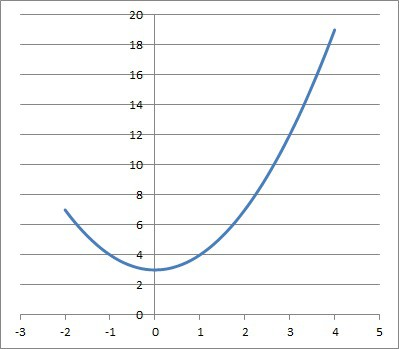The graph of the quadratic function f(x) = x2 + 3 on the domain [-2, 4]. Its maximum is at the right endpoint (4, 17) and its minimum is at the vertex (0, 3).

A quadratic function whose domain is the entire set of real numbers can have only a maximum or a minimum – not both.

### Can A Quadratic Function Open Sideways?

A quadratic function defined by f(x) = ax2 + bx + c cannot open sideways.  It will open upwards (if a > 0, meaning we have a convex or concave up function) or downwards (if a < 0, meaning we have a concave or concave down function).A quadratic function of the form f(x) = ax2 + bx + c cannot open sideways. It can only open up (for a > 0) or down (for a < 0).

However, a quadratic defined by g(y) = ay2 + by + c can open sideways.  For example, the quadratic x = y2 opens sideways (to the right).

### Can A Quadratic Function Have A Negative Exponent?

A quadratic function cannot have negative exponents.  By definition, a quadratic has the form

• f(x) = ax2 + bx + c

This implies that for terms with nonzero coefficients, the exponents of the variable x can be 0, 1, or 2.  There can be no negative exponents in a quadratic function.

### Can A Quadratic Function Have An Inverse?

A quadratic function cannot have an inverse if it is defined on the entire set of real numbers.  This is due to the fact that a quadratic function is not one-to-one on a domain of the set of real numbers.

However, if we restrict its domain, a quadratic function can have a well-defined inverse.  For example, take the quadratic function f(x) = x2 on the set of nonnegative real numbers (domain of x >= 0).

The inverse function would be f-1(x) = √x, defined on the set of nonnegative real numbers (domain of x >= 0).